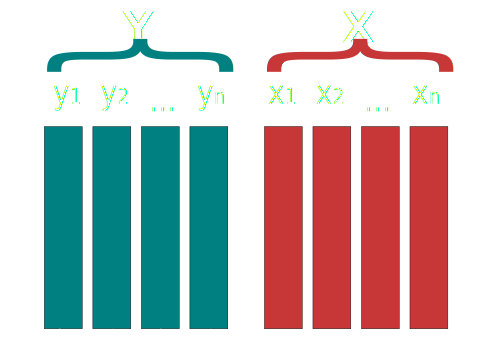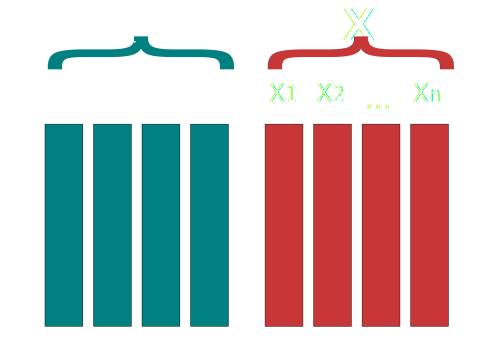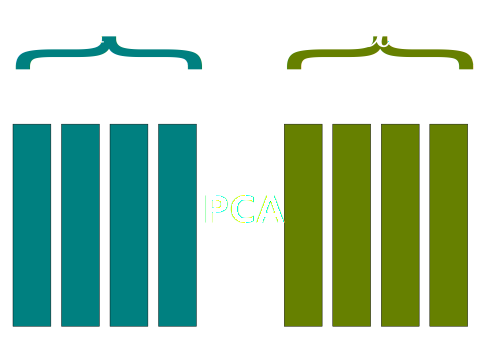# Redundancy analysis (RDA)

## Redundancy analysis

• Simple (unconstrained) ordination analyses (such as PCA) on a single data matrix $$X$$ helps to reveal its major structure (Borcard, Gillet, and Legendre 2011).

• There are not notions of explanatory or response variables.

• On contrary, canonical ordination such as RDA explicitly explores the relationships between two matrices: a response matrix and an explanatory matrix.

## Redundancy analysis

• RDA is the multivariate (meaning multiresponse) technique analogue of regression.

• The method uses a mix of linear regression and principal components analysis (PCA).

• Conceptually, RDA is a multivariate (meaning multiresponse) multiple linear regression followed by a PCA of the table of fitted values.

## Definitions

Lets define :

• $$X$$ a matrix of explanatory variables
• $$Y$$ a matrix of response variables## Definitions

RDA procedure works on both centered matrices. This simply means that that the average of the variable is subtracted from each observation.

$\bar{X}_j = \sum_{i = 1}^{n} X_{ij} = 0$

$\bar{Y}_j = \sum_{i = 1}^{n} Y_{ij} = 0$

## RDA cookbook

These steps are from Borcard, Gillet, and Legendre (2011) which I highly recommend.

1. Regress each (centered) $$y$$ variable on explanatory matrix $$X$$ and compute the fitted ($$\hat{y}$$) and residuals ($$y_{res}$$) vectors.

2. Create a new matrix ($$\hat{Y}$$) containing all the fitted vectors ($$\hat{y}$$).

3. Compute a PCA on $$\hat{Y}$$. This will produces a vector of canonical eigenvalues and a matrix $$U$$ of canonical eigenvectors (principal components).

## Graphical view

$$\hat{Y}$$ is produced using multiple linear regression between $$X$$ and each $$y_i$$.## Graphical view

A PCA is performed on $$\hat{Y}$$ which gives a set of principal component vectors $$U$$.## PCA vs RDA

PCA and RDA are very similar:

• PCA is performed on a matrix with explanatory variables.

• RDA is performed on a matrix of predicted explanatory variables.

## Two types of RDA

Depends on how site scores are calculated (two possibilities).

• $$Y \times U$$ to obtain ordination in the space of the original variables $$Y$$.
• $$\hat{Y} \times U$$ to obtain ordination in the space of the variables $$X$$.

Site scores calculated using $$Y \times U$$ are simply called site scores where as scores calculates using $$\hat{Y} \times U$$ are called site constraints since they are calculated using linear combinations of constraining variables $$X$$.

## Vegan R package

The vegan package makes it very easy to perform RDA in R using the RDA() function.

# Install the package
install.packages("vegan")

library(vegan)

## Vegan R package

#### Basic usage

# X is a matrix or data frame of explanatory variables
my_rda <- rda(Y ~ x1 + x2 + x3, data = X)